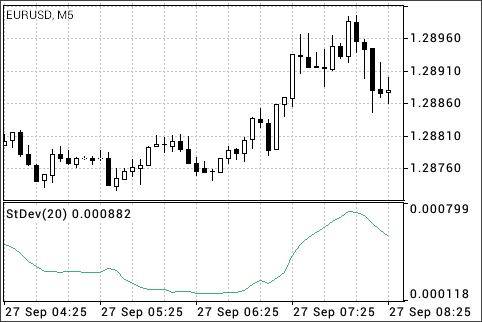# Standard Deviation

Standard Deviation is a value of the market volatility measurement. This indicator describes the range of price fluctuations relative to Moving Average. So, if the value of this indicator is high, the market is volatile, and prices of bars are rather spread relative to the moving average. If the indicator value is low, the market can described as having a low volatility, and prices of bars are rather close to the moving average.

Normally, this indicator is used as a constituent of other indicators. For Bollinger Bands® calculation,

the value of the standard deviation of the symbol is added to its moving average.

The market behavior represents the interchange of high trading activity and languid market. So, the indicator can be interpreted easily:

• if its value is too low, i.e., the market is absolutely inactive, it makes sense to expect a spike soon;
• otherwise, if it is extremely high, it most probably means that activity will decline soon.## Calculation:

StdDev (i) = SQRT (AMOUNT (j = i - N, i) / N)

AMOUNT (j = i - N, i) = SUM ((ApPRICE (j) - MA (ApPRICE , N, i)) ^ 2)

where:

StdDev (i) – Standard Deviation of the current bar;
SQRT – square root;
AMOUNT(j = i - N, i) – the sum of squares from j = i - N to i;
N – the period of smoothing;
ApPRICE (j) – the applied price of the j-th bar;
MA (ApPRICE , N, i) – the value of the N-period moving average on the current bar;
ApPRICE (i) – the applied price of the current bar.